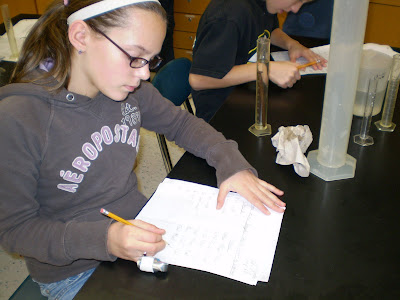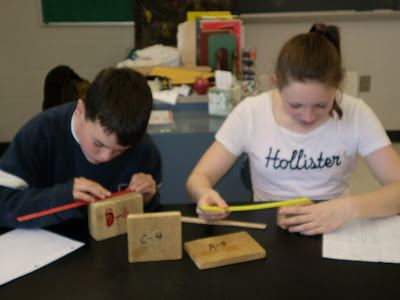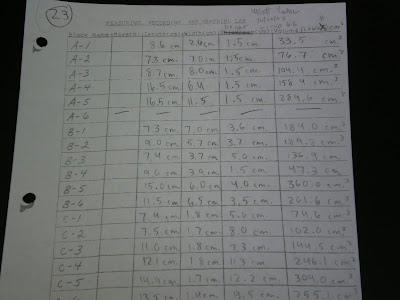## Friday, October 24, 2008

### Volume of a SolidThis week we have been finding the volume of a solid. First, we worked at determining the volume of a regular shaped (rectangular prism) object using the formula V = L x W x H. The units were cubic centimeters (cm3). Using wood blocks and a metric ruler, we measured the length, width, and height of the blocks to the nearest mm. We then used a calculator and multiplied the length times the width times the height and rounded the answer off to the nearest tenth. The units were cm3. We next worked on finding the volume of an irregular shaped object by displacement. We put some water into a graduated cylinder and recorded it's volume. We then lowered our object into the water and the water level went up. We recorded this new level of water and then subtracted the original amount of water from the new amount of water. The result was the volume of the object placed into the water. The units were cm3 or ml.